# NCERT Solutions class-11 Maths Miscellaneous## myCBSEguide App

CBSE, NCERT, JEE Main, NEET-UG, NDA, Exam Papers, Question Bank, NCERT Solutions, Exemplars, Revision Notes, Free Videos, MCQ Tests & more.

Miscellaneous Exercise

1. Write the negation of the following statements:

(i)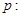For every positive real number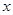, the number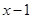is also positive.

(ii)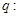All cats scratch.

(iii)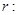For every real number, either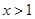or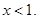(iv)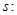There exists a numbersuch that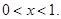Ans. (i)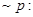There exists a positive real numbersuch thatis not positive.

(ii)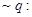There exists a cat which does not scratch.

(iii)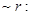There exists a real numbersuch thator(iv)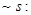There does not exist a numbersuch that2. State the converse and contrapositive of each of the following statements:

(i)A positive integer is prime only if it has no divisors other than 1 and itself.

(ii)I go to a beach whenever it is a sunny day.

(iii)If it is not outside, then you feel thirsty.

Ans. (i) Contrapositive: If a positive integer has divisors other than 1 and itself then it is not prime.

Converse: If a positive integer has no divisors other than 1 and itself then it is a prime.

(ii) Contrapositive: If I do not go to a beach then it is not a sunny day.

Converse: If I go to a beach then it is a sunny day.

(iii) Contrapositive: If you do not feel thirsty then it is not hot outside.

Converse: If you feel thirsty then it is hot outside.

3. Rewrite each of the following statements in the form “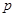it and only if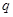”.

(i)It is necessary to have a password to log on to the server.

(ii)There is traffic Jam whenever it rains.

(iii)You can access the website only if you pay a subscription fee.

Ans. (i) If you log on to the server then you have a password.

(ii) If it rains, then there is traffic jam.

(iii) If you can access the website, then you pay a subscription fee.

4. Rewrite each of the following statements in the form “it and only if”.

(i)If you watch television, then your mind is free and if your mind is free, then you watch television.

(ii)For you to get an A grade, it is necessary and sufficient that you do all the homework regularly.

(iii)If a quadrilateral is equiangular, then it is a rectangle and if a quadrilateral is a rectangle, then it is equiangular.

Ans. (i) You watch television if and only if your mind is free.

(ii) You get an A grade if and only if you do all the homework regularly.

(iii) A quadrilateral is equiangular if and only if it is a rectangle.

5. Given below are two statements:25 is a multiple of 5.25 is a multiple of 8.

Write the compound statements connecting these two statements with “and” and “or”. In both cases check the validity of the compound statement.

Ans. The compound statement with “and” is: 25 is a multiple of 5 and 8.

Since,is true andis false therefore the compound statement with “and” is not true.

Therefore, the statement “and” is not valid.

Now the compound statement with “or” is: 25 is a multiple of 5 or 8.

Since,is true andis false therefore the compound statement with “or” is true.

Therefore, the statement “and” is valid.

6. Check the validity of the statements given below by the method given against it:

(i)The sum of an irrational number and a rational number is irrational (by contradiction method)

(ii)If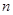is a real number with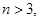then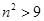(by contradiction method)

Ans. (i) Let us assume thatis not true.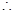Sum of an irrational and a rational number is not irrational.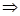There exists an irrational number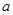and a rational number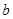such that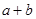is not irrational.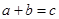(say) is a rational number.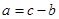is rational.

Butis irrational, which is contradiction, therefore our supposition is wrong.

Therefore,is true.

(ii) Let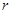and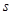be the statements given by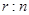is a real number with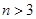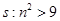If possible letis not true, then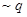is true.and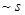is true.is a real number withand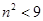which is contradiction, therefore our supposition is wrong.

Therefore,is true.

7. Write the following statement in five different ways, conveying the same meaning:If a triangle is equiangular, then it is an obtuse angled triangle.

Ans. (i) A triangle is equiangular implies that it is an obtuse angled triangle.

(ii) A triangle is equiangular only if it an obtuse angled triangle.

(iii) For a triangle to be equiangular it is necessary it is an obtuse angled triangle.

(iv) For a triangle to be obtuse angled triangle it is sufficient that the triangle is equiangular.

(v) Is a triangle is not equiangular then it is not an obtuse angled triangle.